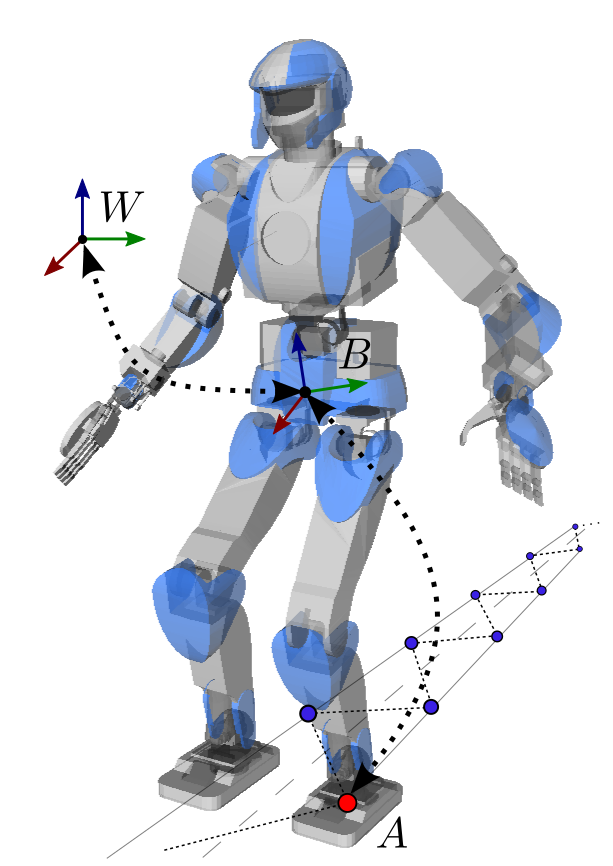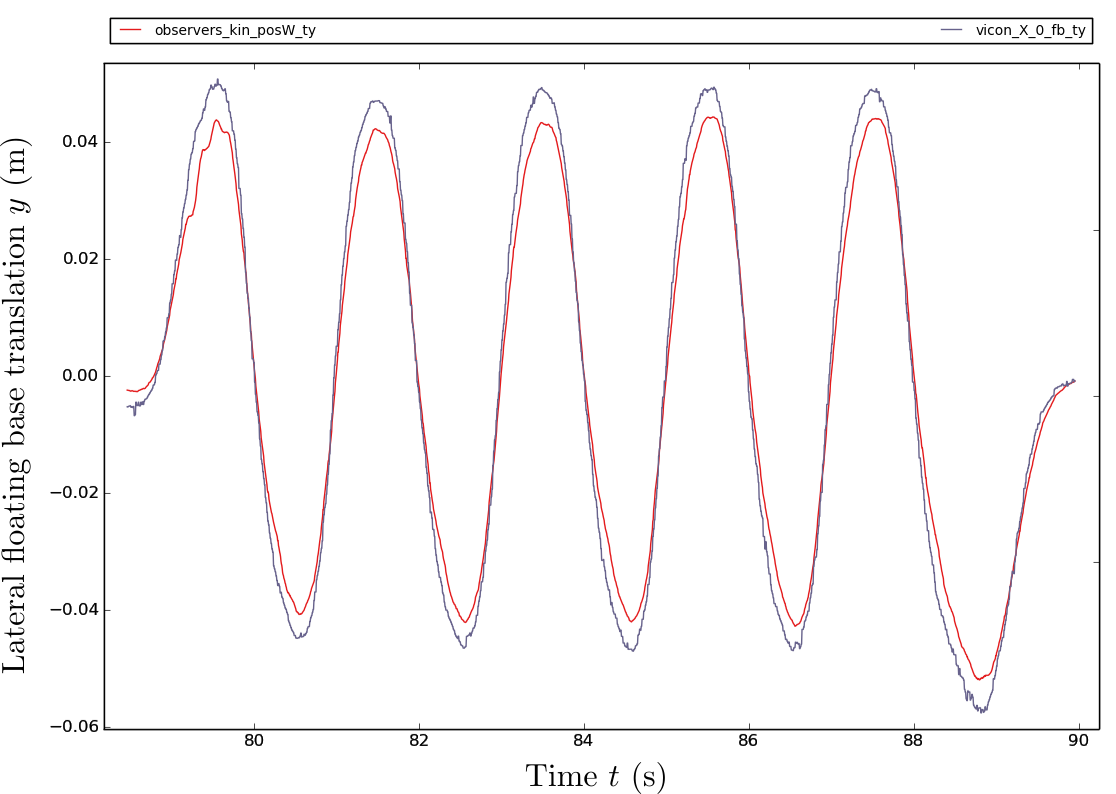# Floating base estimation

For a legged system, locomotion is about controlling the unactuated floating base to a desired location in the world. Before a control action can be applied, the first thing is to estimate the position and orientation of this floating base. In what follows, we will see the simple anchor point solution, which is a good way to get started and works well in practice. For example, it is the one used in this stair climbing experiment.

## Orientation of the floating base

We assume that our legged robot is equipped with an inertial measurement unit (IMU) in its pelvis link, and attach the base frame $$B$$ of our floating base to the same link. The IMU contains gyroscopes, providing angular velocity measurements $${}^B\bfomega_B$$, and accelerometers, providing linear acceleration measurements $${}^B\bfa$$ that include both gravity and linear accelerations caused by robot motions. These measurements can be used to estimate the orientation $${}^W\bfR_B$$ of the floating base with respect to an inertial world frame $$W$$, but only partially. Let us outline how this process works.

### Integration of angular velocities

Define the world frame as the initial floating base of the system, so that $$\bfR^i_0 := {}^W\bfR^i_B = \bfI_3$$ with $$\bfI_3$$ the $$3 \times 3$$ identity matrix. At discrete time $$t_k$$, gyroscopes measure the angular velocity $${}^B \bfomega_B(t_k)$$ of the floating base $$B$$ relative to the inertial frame, expressed in $$B$$. The discrete series $$\bfomega_k := {}^B \bfomega_B(t_k)$$ of angular velocity measurements can be integrated to get:

\begin{equation*} \bfR^i_{k+1} = \bfR^i_{k} \exp([\bfomega_k \times] \Delta t) \end{equation*}

with $$\Delta t = t_k - t_{k-1}$$ the duration between two measurements and $$[\bfomega_k \times]$$ the skew-symmetric matrix formed from the angular velocity vector. In practice the matrix exponential can be expanded by Rodrigues' rotation formula:

\begin{equation*} \bfR^i_{k+1} = \bfR^i_{k} \left(\bfI_3 + \frac{\sin \theta_k}{\theta_k} [\bfomega_k \times] + \frac{1 - \cos \theta_k}{\theta_k^2} [\bfomega_k \times]^2 \right) \end{equation*}

with $$\theta_k = \| \omega_k \| \Delta t$$. This integration scheme gives us a first estimate of the orientation of the floating base over time, however it quickly drifts in practice because gyroscopes have a (time-varying) measurement bias.

### Accelerometer-based estimation

Accelerometers can be used to provide an alternative estimate of the floating base orientation. When the robot is not moving, what these accelerometers see on average is the gravity vector $${}^B\bfg$$ expressed in $$B$$. As the gravity vector $${}^W\bfg \approx [0 \ 0 \ {-9.81}]$$ in the inertial frame is known, we can estimate $$\bfR^g = {}^W \bfR_B$$ as the rotation matrix that sends $${}^B \bfg$$ onto $${}^W \bfg$$. Let us denote by $${}^B \bfu$$ and $${}^W \bfu$$ the normalized vectors from $${}^B \bfg$$ and $${}^W \bfg$$ respectively:

\begin{equation*} \begin{array}{rcl} \bfv & = & {}^B \bfu \times {}^W \bfu \\ c & = & {}^B \bfu \cdot {}^W \bfu \\ \bfR^g & = & \bfI_3 + [\bfv \times] + \frac{1}{1 + c} [\bfv \times]^2 \end{array} \end{equation*}

This formula performs well to estimate the roll and pitch angles of the rotation $${}^W \bfR_B$$ of the floating base, however it cannot estimate the third yaw component because the gravity vector is invariant by rotation around the yaw axis. Basically, at our levels of perception, gravity is felt the same whether you face north, east or south.

### Drift compensation of the gyroscope bias

The drawbacks of these two estimates fortunately appear at different frequencies: angular-velocity integration drifts in the long run, accelerometer-based estimation is perturbed in the short run as the robot moves. A common solution is then to rely mostly on the former, compensating its drift by low-frequency feedback of the latter. On the HRP-4 humanoid, this solution is implemented in an extended Kalman filter that runs on the robot's embedded computer. It can also be realized by a complementary filter such as (Madgwick, 2010), which provides remarkable C source code in its Appendix.

## Translation of the floating base

Now that we have estimated the orientation $${}^W\bfR_B$$ of the floating base with respect to the world frame, let us estimate its translation $${}^W \bfp_B$$ using the anchor point strategy. Its idea is summed up in the following figure:We select an anchor point $$A$$ at a fixed location on the foot sole, for instance the point closest to the ankle frame (a pertinent choice for Honda and HRP robots to limit deflection errors caused by their rubber foot flexibility), and assume that this point is in contact at the position $${}^W \bfp_A$$ on the ground where it is planned to be in the world frame. In the floating-base frame, the position $${}^B \bfp_A$$ of this point is known by forward kinematics. From the anchor-point assumption, we can then compute the floating-base translation by:

\begin{equation*} {}^W \bfp_A = {}^W \bfp_B + {}^W \bfR_B {}^B \bfp_A \ \Longrightarrow \ {}^W \bfp_B = {}^W \bfp_A - {}^W \bfR_B {}^B \bfp_A \end{equation*}

This estimator is simple to implement and does not require additional parameters. While walking, the anchor point can be chosen (1) closest to the ankle frame during single-support phases and (2) continuously varying from one ankle frame to the other during double-support phases. Continuous variations help avoid discontinuities, which are generally undesirable in a controller.

While simple to implement, this estimator performs quite well in practice. Here is for example a comparison to more precise VICON measurements ran while HRP-4 was stepping in place:In this example, the estimation error in the lateral direction was at most 0.8 cm.

## Divergent component of motion

For walking stabilization, the controller may need to estimate not only the floating base position but also the divergent component of motion (DCM) $$\bfxi$$, which depends on the center of mass (CoM) position $$\bfc$$ and velocity $$\bfcd$$ by:

\begin{equation*} \bfxi = \bfc + \frac{\bfcd}{\omega} \end{equation*}

where $$\omega = \sqrt{g / h}$$ is a known constant. For a straightforward solution, the CoM position can be derived from the floating base and joint encoder measurements by forward kinematics. Its velocity is then obtained via a first-order low-pass filter with cutoff period $$T_\textit{cutoff} = 10~\text{ms}$$:

\begin{equation*} \alpha = \frac{\Delta t}{T_\textit{cutoff}} \ \Longrightarrow \ \bfcd_{k+1} = \alpha \frac{\bfc_{k+1} - \bfc_k}{\Delta t} + (1 - \alpha) \bfcd_k \end{equation*}

where $$\Delta t$$ is the duration of a control cycle. The DCM is finally computed from the CoM position $$\bfc_k$$ and velocity $$\bfcd_k$$. While simple to implement, this scheme is the one we applied in a walking and stair climbing controller for the HRP-4 humanoid.

## To go further

Estimating the floating base orientation from IMU measurements is a key component in the above process, of which we only gave the outline. To go further, you can take a look at Sections III and V of (Forster et al., 2016).

### Kalman and complementary filters

There is significant literature on the topic of floating base estimation, and more generally state estimation for legged robots. One way is to design a Kalman or complementary filter. The following open source libraries follow this approach:

• Pronto: an extended Kalman filter for floating base estimation, used by the MIT DRC team during the DARPA Robotics Challenge.
• SEROW: a Kalman filter for floating base and CoM position and velocity estimation.
• state-observation: a collection of Kalman filters based on different contact assumptions.

### Numerical optimization

Another approach relies on quadratic programming to minimize model and measurement errors. Quadratic programming can also be used to fuse simple estimators with time-varying weights. The SpringEstimator library follows this approach. It applies a nonlinear hierarchical optimization that can estimate multiple compliant links in contact. Technical details are provided around Fig. 8 of this paper.

© Stéphane Caron — Pages of this website are under the Creative Commons CC BY 4.0 license.